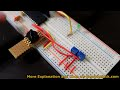### Using the Slope Intercept Formula to Adjust the ADC Value for Trimmer Potentiometers Showing on the LCD

The trimmer potentiometers are used to adjust the high and low thresholds for the pressure sensor circuit. The adjustment needs to read between 0 and 33 rather than the ADC result of 0 and 1023, so the slope intercept formula is a good formula to use in this case.
y = mx + b

f(x) = y or f(x) = mx + b

f(0) = 0
f(1023) = 33

m = rise / run = ?y/?x
= (0 - 33) / (0 - 1023)
= -33 / -1023
= 0.0323

To find b (plug in x and y values, the easiest values in this case would be 0,0):

y = mx + b
0 = 0.0323(0) + b
0 = 0 + b

b = 0

So the formula is:

y = 0.0323(x)

where